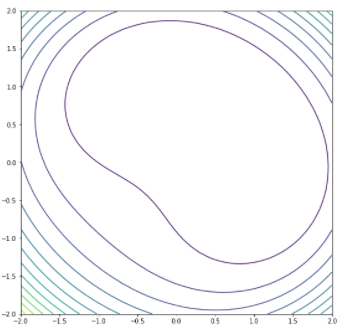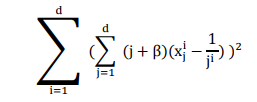top of page
Search

# Python Implementation of Perm 0,d beta Function

Updated: Jul 18, 2021Mathematical DefinitionInput Domain

It can be defined into any input domain but usually perm 0, d, beta function evaluated on the hypercube 𝑥𝑖 𝜖 [−𝑑, 𝑑], for all 𝑖 = 1, … . , 𝑑.

Global Minima

The function has one global minimum 𝑓(𝑥 ∗) = 0, 𝑎𝑡 𝑥 ∗ = (1, 1 /2 , … , 1 /𝑑 )

Description and Features

The function is defined on 2- dimensional space

Python Implementation

```% Please forward any comments or bug reports in chat
Copyright 2021. INDUSMIC PRIVATE LIMITED.THERE IS NO WARRANTY, EXPRESS OR IMPLIED. WE DO NOT ASSUME ANY LIABILITY FOR THE USE OF THIS PROGRAM. If software is modified to produce derivative works, such modified software should be clearly marked. Additionally, user can redistribute it and/or modify it under the terms of the GNU General Public License. This program is distributed in the hope that it will be useful, but WITHOUT ANY WARRANTY. See the GNU General Public License for more details.
% for any support connect with us on help.indusmic@gmail.com
% Author: Ekta Kumari

import matplotlib.pyplot as plt
import numpy as np
from numpy import sin

from numpy import e
from numpy import meshgrid
from mpl_toolkits.mplot3d import Axes3D
from matplotlib import cm

def f(x1,x2):

return (11*(x1-1)+12*(x2-0.5))**2 + (11*(x1**2-1)+12*(x2**2-0.25))**2

x1=np.linspace(-2,2)
x2=np.linspace(-2,2)
r_min,r_max=1,2

x1,x2=np.meshgrid(x1,x2)
results=f(x1,x2)

figure=plt.figure(figsize=(9,9))
axis=figure.gca(projection='3d')
axis.contour3D(x1, x2, results,450)
axis.set_title('Perm function 0,d,beta')
axis.view_init(40,40)
axis.set_xlabel('X')
axis.set_ylabel('Y')
axis.set_zlabel('Z')
plt.show()```

References:

 Jamil, Momin, and Xin-She Yang. "A literature survey of benchmark functions for global optimization problems." International Journal of Mathematical Modelling and Numerical Optimization 4.2 (2013): 150-194.